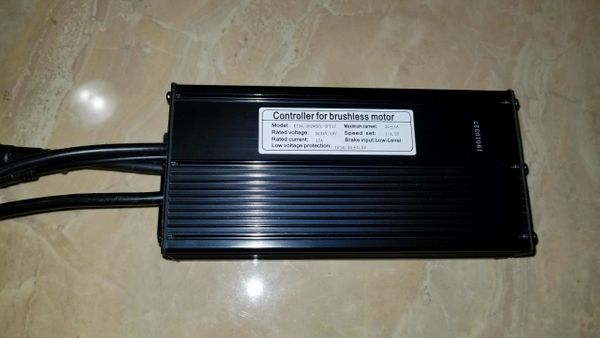\$129.00

#### Dimensions are 7.2 x 3.2 x 1.7in.  This controller is required to be use with our color display KT-LCD8H. You can NOT use the Rad's LCD display with our controllers and vice versa.

Use these LCD settings for the Rad bike and watch the video link below on how to get into the settings.

P1 = 100

P2 = 5

P3 = 0

P4 = 0

P5 = 15

C1 = 3

C2 = 0

C3 = 8

C4 = 3

C5 = 10

C6 = 3

C7 = 1

C8 = 0

C9 = 0

C10 = n

C11 = 0

C12 = 4

C13 = 0

C14 = 2